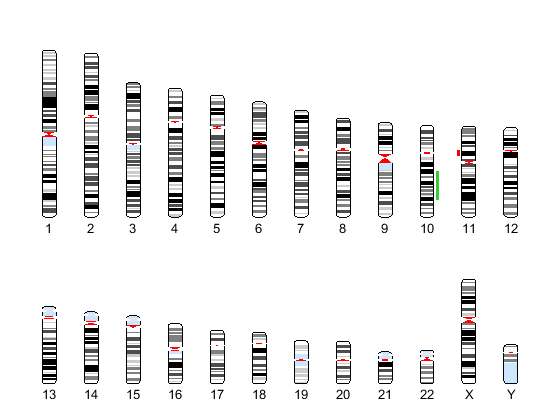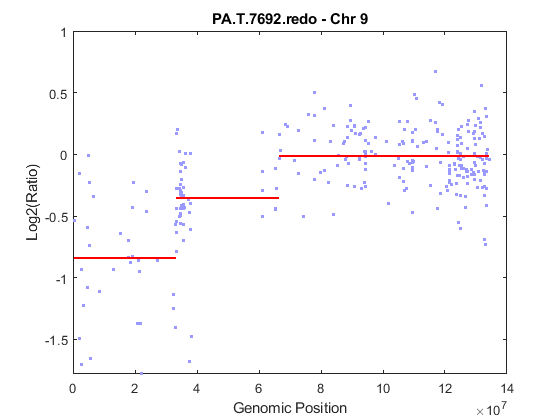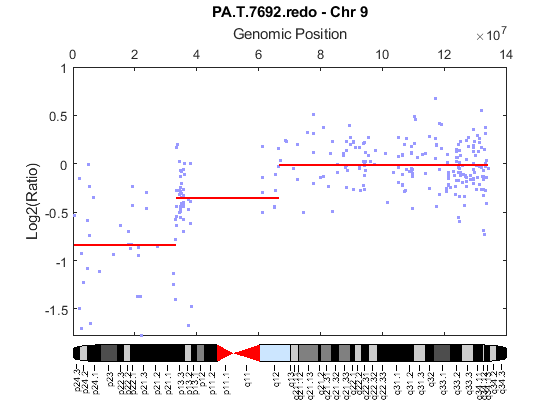# cghcbs

Perform circular binary segmentation (CBS) on array-based comparative genomic hybridization (aCGH) data

## Syntax

```SegmentStruct = cghcbs(CGHData) SegmentStruct = cghcbs(CGHData, ...'Alpha', AlphaValue, ...) SegmentStruct = cghcbs(CGHData, ...'Permutations', PermutationsValue, ...) SegmentStruct = cghcbs(CGHData, ...'Method', MethodValue, ...) SegmentStruct = cghcbs(CGHData, ...'StoppingRule', StoppingRuleValue, ...) SegmentStruct = cghcbs(CGHData, ...'Smooth', SmoothValue, ...) SegmentStruct = cghcbs(CGHData, ...'Prune', PruneValue, ...) SegmentStruct = cghcbs(CGHData, ...'Errsum', ErrsumValue, ...) SegmentStruct = cghcbs(CGHData, ...'WindowSize', WindowSizeValue, ...) SegmentStruct = cghcbs(CGHData, ...'SampleIndex', SampleIndexValue, ...) SegmentStruct = cghcbs(CGHData, ...'Chromosome', ChromosomeValue, ...) SegmentStruct = cghcbs(CGHData, ...'Showplot', ShowplotValue, ...) SegmentStruct = cghcbs(CGHData, ...'Verbose', VerboseValue, ...) ```

## Input Arguments

 `CGHData` Array-based comparative genomic hybridization (aCGH) data in either of the following forms:Structure with the following fields:`Sample` — Cell array of character vectors or string vector containing the sample names (optional).`Chromosome` — Vector containing the chromosome numbers on which the clones are located.`GenomicPosition` — Vector containing the genomic positions (in any unit) to which the clones are mapped.`Log2Ratio` — Matrix containing log2 ratio of test to reference signal intensity for each clone. Each row corresponds to a clone, and each column corresponds to a sample.Matrix in which each row corresponds to a clone. The first column contains the chromosome number, the second column contains the genomic position, and the remaining columns each contain the log2 ratio of test to reference signal intensity for a sample. `AlphaValue` Scalar that specifies the significance level for the statistical tests to accept change points. Default is `0.01`. `PermutationsValue` Scalar that specifies the number of permutations used for p-value estimation. Default is `10,000`. `MethodValue` Character vector or string that specifies the method to estimate the p-values. Choices are `'Perm'` or `'Hybrid'` (default). `'Perm'` does a full permutation, while `'Hybrid'` uses a faster, tail probability-based permutation. When using the `'Hybrid'` method, the `'Perm'` method is applied automatically when segment data length becomes less than 200. `StoppingRuleValue` Controls the use of a heuristic stopping rule, based on the method described by Venkatraman and Olshen (2007), to declare a change without performing the full number of permutations for the p-value estimation, whenever it becomes very likely that a change has been detected. Choices are `true` or `false` (default).TipSet this property to `true` to increase processing speed. Set this property to `false` to maximize accuracy. `SmoothValue` Controls the smoothing of outliers before segmenting using the procedure explained by Olshen et al. (2004). Choices are `true` (default) or `false`. `PruneValue` Controls the elimination of change points identified due to local trends in the data that are not indicative of real copy number change, using the procedure explained by Olshen et al. (2004). Choices are `true` or `false` (default). `ErrsumValue` Scalar that specifies the allowed proportional increase in the error sum of squares when eliminating change points using the `'Prune'` property. Commonly used values are `0.05` and `0.1`. Default is `0.05`. `WindowSizeValue` Scalar that specifies the size of the window (in data points) used to divide the data when using the `'Perm'` method on large data sets. Default is `200`. `SampleIndexValue` A single sample index or a vector of sample indices that specify the sample(s) to analyze. Default is all sample indices. `ChromosomeValue` A single chromosome number or a vector of chromosome numbers that specify the data to analyze. Default is all chromosome numbers. `ShowplotValue` Controls the display of plots of the segment means over the original data. Choices are either: `true` — All chromosomes in all samples are plotted. If there are multiple samples in `CGHData`, then each sample is plotted in a separate Figure window. `false` — No plot.`W` — The layout displays all chromosomes in the whole genome in one plot in the Figure window.`S` — The layout displays each chromosome in a subplot in the Figure window.`I` — An integer specifying only one of the chromosomes in `CGHData` to be plotted.Default is:`false` — When return values are specified.`true` and `W` — When return values are not specified. `VerboseValue` Controls the display of a progress report of the analysis. Choices are `true` (default) or `false`.

## Output Arguments

 `SegmentStruct` Structure containing segmentation information in the following fields:`Sample` — Sample name from `CGHData` input argument. If the input argument does not include sample names, then sample names are assigned as `Sample1`, `Sample2`, and so forth.`SegmentData` — Structure array containing segment data for the sample in the following fields:`Chromosome` — Chromosome number on which the segment is located.`Start` — Genomic position at the start of the segment (in the same units as used for the `CGHData` input).`End` — Genomic position at the end of the segment (in the same units as used for the `CGHData` input).`Mean` — Mean value of the log2 ratio of the test to reference signal intensity for the segment.

## Description

`SegmentStruct = cghcbs(CGHData)` performs circular binary segmentation (CBS) on array-based comparative genomic hybridization (aCGH) data to determine the copy number alteration segments (neighboring regions of DNA that exhibit a statistical difference in copy number) and change points.

Note

The CBS algorithm recursively splits chromosomes into segments based on a maximum t statistic estimated by permutation. This computation can be time consuming. If `n` = number of data points, then computation time ~ O(`n`2).

```SegmentStruct = cghcbs(CGHData, ...'PropertyName', PropertyValue, ...)``` calls `cghcbs` with optional properties that use property name/property value pairs. You can specify one or more properties in any order. Each `PropertyName` must be enclosed in single quotation marks and is case insensitive. These property name/property value pairs are as follows:

``` SegmentStruct = cghcbs(CGHData, ...'Alpha', AlphaValue, ...)``` specifies the significance level for the statistical tests to accept change points. Default is `0.01`.

```SegmentStruct = cghcbs(CGHData, ...'Permutations', PermutationsValue, ...)``` specifies the number of permutations used for p-value estimation. Default is `10,000`.

```SegmentStruct = cghcbs(CGHData, ...'Method', MethodValue, ...)``` specifies the method to estimate the p-values. Choices are `'Perm'` or `'Hybrid'` (default). `'Perm'` does a full permutation, while `'Hybrid'` uses a faster, tail probability-based permutation. When using the `'Hybrid'` method, the `'Perm'` method is applied automatically when segment data length becomes less than 200.

```SegmentStruct = cghcbs(CGHData, ...'StoppingRule', StoppingRuleValue, ...)``` controls the use of a heuristic stopping rule, based on the method described by Venkatraman and Olshen (2007), to declare a change without performing the full number of permutations for the p-value estimation, whenever it becomes very likely that a change has been detected. Choices are `true` or `false` (default).

```SegmentStruct = cghcbs(CGHData, ...'Smooth', SmoothValue, ...)``` controls the smoothing of outliers before segmenting, using the procedure explained by Olshen et al. (2004). Choices are `true` (default) or `false`.

```SegmentStruct = cghcbs(CGHData, ...'Prune', PruneValue, ...)``` controls the elimination of change points identified due to local trends in the data that are not indicative of real copy number change, using the procedure explained by Olshen et al. (2004). Choices are `true` or `false` (default).

```SegmentStruct = cghcbs(CGHData, ...'Errsum', ErrsumValue, ...)``` specifies the allowed proportional increase in the error sum of squares when eliminating change points using the `'Prune'` property. Commonly used values are `0.05` and `0.1`. Default is `0.05`.

```SegmentStruct = cghcbs(CGHData, ...'WindowSize', WindowSizeValue, ...)``` specifies the size of the window (in data points) used to divide the data when using the `'Perm'` method on large data sets. Default is `200`.

```SegmentStruct = cghcbs(CGHData, ...'SampleIndex', SampleIndexValue, ...)``` analyzes only the sample(s) specified by `SampleIndexValue`, which can be a single sample index or a vector of sample indices. Default is all sample indices.

```SegmentStruct = cghcbs(CGHData, ...'Chromosome', ChromosomeValue, ...)``` analyzes only the data on the chromosomes specified by `ChromosomeValue`, which can be a single chromosome number or a vector of chromosome numbers. Default is all chromosome numbers.

```SegmentStruct = cghcbs(CGHData, ...'Showplot', ShowplotValue, ...)``` controls the display of plots of the segment means over the original data. Choices are `true`, `false`, `W`, `S`, or `I`, an integer specifying one of the chromosomes in `CGHData`. When `ShowplotValue` is `true`, all chromosomes in all samples are plotted. If there are multiple samples in `CGHData`, then each sample is plotted in a separate Figure window. When `ShowplotValue` is `W`, the layout displays all chromosomes in one plot in the Figure window. When `ShowplotValue` is `S`, the layout displays each chromosome in a subplot in the Figure window. When `ShowplotValue` is `I`, only the specified chromosome is plotted. Default is either:

• `false` — When return values are specified.

• `true` and `W` — When return values are not specified.

```SegmentStruct = cghcbs(CGHData, ...'Verbose', VerboseValue, ...)``` controls the display of a progress report of the analysis. Choices are `true` (default) or `false`.

## Examples

collapse all

Analyze data from the Coriell cell line study

Load the array-based CGH (aCGH) data from the Coriell cell line study (Snijders, A. et al., 2001).

```load coriell_baccgh ```

Analyze all chromosomes of sample 3 (GM05296) of the aCGH data and return segmentation data in a structure, S. Plot the segment means over the original data for all chromosomes of this sample.

```S = cghcbs(coriell_data,'sampleindex',3,'showplot',true); ```
```Analyzing: GM05296. Current chromosome 1 Analyzing: GM05296. Current chromosome 2 Analyzing: GM05296. Current chromosome 3 Analyzing: GM05296. Current chromosome 4 Analyzing: GM05296. Current chromosome 5 Analyzing: GM05296. Current chromosome 6 Analyzing: GM05296. Current chromosome 7 Analyzing: GM05296. Current chromosome 8 Analyzing: GM05296. Current chromosome 9 Analyzing: GM05296. Current chromosome 10 Analyzing: GM05296. Current chromosome 11 Analyzing: GM05296. Current chromosome 12 Analyzing: GM05296. Current chromosome 13 Analyzing: GM05296. Current chromosome 14 Analyzing: GM05296. Current chromosome 15 Analyzing: GM05296. Current chromosome 16 Analyzing: GM05296. Current chromosome 17 Analyzing: GM05296. Current chromosome 18 Analyzing: GM05296. Current chromosome 19 Analyzing: GM05296. Current chromosome 20 Analyzing: GM05296. Current chromosome 21 Analyzing: GM05296. Current chromosome 22 Analyzing: GM05296. Current chromosome 23 Analyzing: GM05296. Current chromosome 26 Analyzing: GM05296. Current chromosome 27 Analyzing: GM05296. Current chromosome 30 ```Chromosome 10 shows a gain, while chromosome 11 shows a loss.

Display copy number alteration regions aligned to a chromosome ideogram

Create a structure containing segment gain and loss information for chromosomes 10 and 11 from sample 3, making sure the segment data is in bp units. (You can determine copy number variance (CNV) information by exploring S, the structure of segments returned by the cghcbs function. For the `'CNVType'` field, use 1 to indicate a loss and 2 to indicate a gain.

```cnvStruct = struct('Chromosome', [10 11],... 'CNVType', [2 1],... 'Start', [S.SegmentData(10).Start(2),... S.SegmentData(11).Start(2)]*1000,... 'End', [S.SegmentData(10).End(2),... S.SegmentData(11).End(2)]*1000) ```
```cnvStruct = struct with fields: Chromosome: [10 11] CNVType: [2 1] Start: [66905000 35416000] End: [110412000 43357000] ```

Pass the structure to the `chromosomeplot` function using the `'CNV'` option to display the copy number gains (green) and losses (red) aligned to the human chromosome ideogram. Specify kb units for the display of segment information in the data tip.

```chromosomeplot('hs_cytoBand.txt', 'CNV', cnvStruct, 'unit', 2) ```Analyze data from a pancreatic cancer study

Load the aCGH data from a pancreatic cancer study (Aguirre, A. et al., 2004).

```load pancrea_oligocgh ```

Analyze only chromosome 9 in sample 32 of the CGH data and return the segmentation data in a structure, PS. Plot the segment means over the original data for chromosome 9 in this sample.

```PS = cghcbs(pancrea_data,'sampleindex',32,'chromosome',9,... 'showplot',9); ```
```Analyzing: PA.T.7692.redo. Current chromosome 9 ```Chromosome 9 contains two segments that indicate losses. For more detailed information on interpreting the data, see Aguirre, A. et al. (2004).

Use the `chromosomeplot` function with the `'addtoplot'` option to add the ideogram of chromosome 9 for Homo sapiens to the plot of the segmentation data.

```chromosomeplot('hs_cytoBand.txt', 9, 'addtoplot', gca) ```## References

 Olshen, A.B., Venkatraman, E.S., Lucito, R., and Wigler, M. (2004). Circular binary segmentation for the analysis of array-based DNA copy number data. Biostatistics 5, 4, 557–572.

 Venkatraman, E.S., and Olshen, A.B. (2007). A Faster Circular Binary Segmentation Algorithm for the Analysis of Array CGH Data. Bioinformatics 23(6), 657–663.

 Venkatraman, E.S., and Olshen, A.B. (2006). DNAcopy: A Package for Analyzing DNA Copy Data. `https://www.bioconductor.org/packages/2.1/bioc/html/DNAcopy.html`

 Snijders, A.M., Nowak, N., Segraves, R., Blackwood, S., Brown, N., Conroy, J., Hamilton, G., Hindle, A.K., Huey, B., Kimura, K., Law, S., Myambo, K., Palmer, J., Ylstra, B., Yue, J.P., Gray, J.W., Jain, A.N., Pinkel, D., and Albertson, D.G. (2001). Assembly of microarrays for genome-wide measurement of DNA copy number. Nature Genetics 29, 263–264.

 Aguirre, A.J., Brennan, C., Bailey, G., Sinha, R., Feng, B., Leo, C., Zhang, Y., Zhang, J., Gans, J.D., Bardeesy, N., Cauwels, C., Cordon-Cardo, C., Redston, M.S., DePinho, R.A., and Chin, L. (2004). High-resolution characterization of the pancreatic adenocarcinoma genome. PNAS 101, 24, 9067–9072.

## Version History

Introduced in R2007b